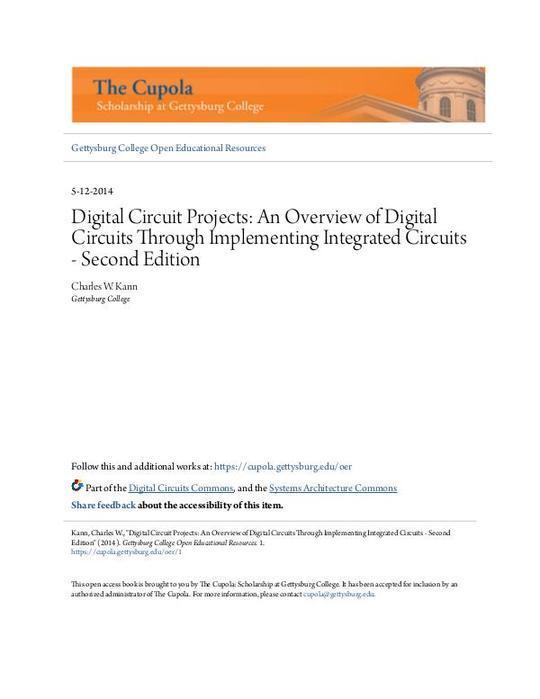Free

# Digital Circuit Projects: An Overview of Digital Circuits Through Implementing Integrated Circuits - Second Edition

By Unknown
Free
Book Description
• 5-12-2014
• Digital Circuit Projects: An Overview of Digital Circuits Through Implementing Integrated Circuits - Second Edition
• Charles W. Kann
• Digital Circuit Projects: An Overview of Digital Circuits Through Implementing Integrated Circuits - Second Edition
• Description
• Keywords
• Disciplines
• I Forward
• I.1 Why this book?
• I.2 Intended Audience
• I.3 Easy to understand circuit design and implementation
• I.4 Material covered in the text
• III About the second edition
• IV Using this book in a class on Computer Organization
• Chapter 1 Before you start
• 1.1 Introduction
• 1.2 Computers and magic
• 1.
• 1.4 Materials Needed
• 1.4.1 Logisim
• 1.4.2 Hardware
• Chips
• Tools
• Miscellaneous
• 1.5 Some notes
• 1.6 Conclusion
• Chapter 2 Background Material
• 2.1 Introduction
• 2.2 A Universal Set of Boolean Operations
• 2.3 Truth Tables
• 2.4 Disjunctive Normal Form(DNF)
• 2.4.1 Boolean Relationships
• 2.5 Karnaugh Maps (K-maps)
• 2.5.1 Gray Codes
• 2.5.2 2-Variable Karnaugh Maps
• 2.5.3 3-Variable Karnaugh Maps
• 2.5.4 4-Variable Karnaugh Maps
• 2.5.5 Don’t care conditions
• 2.6 Conclusion
• 2.7 Exercises
• Chapter 3 Getting started
• 3.1 Introduction
• 3.2 Logisim circuit to turn on a light
• 3.3 Implementing the switch circuit to turn on a light
• 3.3.2 Stripping wires
• 3.3.3 Powering the Circuit
• 3.3.4 Installing the switch
• 3.3.5 Completing the Circuit
• 3.4 Debugging the circuit
• 3.5 Exercises
• Chapter 4 Gates
• 4.1 Introduction
• 4.2 Boolean logic and binary values
• 4.3 Unary operations
• 4.4 Binary Operations
• 4.5 Implementing the AND gate circuit
• 4.5.1 ICs and the 7408 chip
• 4.5.2 The datasheet
• 4.5.3 Creating the AND circuit
• 4.6 Exercises
• Chapter 5 Associative Boolean operators
• 5.1 Introduction
• 5.2 Modeling associative operations in Logisim
• 5.3 Implementing the circuit
• 5.3.1 Implementing the serial AND circuit
• 5.3.2 Implementing the parallel AND circuit
• 5.4 Conclusion
• 5.5 Exercises
• 6.1 Introduction
• 6.5 Conclusion
• 6.6 Exercises
• Chapter 7 Decoders
• 7.1 Introduction
• 7.2 Decoder circuit
• 7.3 2-to-4 decoder implementation
• 7.4 Implementing a decoder using a single chip
• 7.4.1 The 74139 chip
• 7.4.2 Implementing one 2-to-4 decoder using the 74139 chip
• 7.5 Conclusion
• 7.6 Exercises
• Chapter 8 Multiplexers
• 8.1 Introduction
• 8.2 Circuit Diagram for a MUX
• 8.3 Implementing a MUX
• 8.4 74153 MUX chip
• 8.5 74153 circuit diagram
• 8.6 Implementing the 74153 circuit
• 8.7 Conclusion
• 8.8 Exercises
• Chapter 9 Memory basics - flip-flops and latches
• 9.1 Introduction
• 9.2 Background material
• 9.2.1 State
• 9.2.2 Static and dynamic memory
• 9.2.3 Square Wave
• 9.3 Latches
• 9.3.1 D latch
• 9.3.2 Circuit diagram for a D latch
• 9.3.3 Implementing the D latch
• 9.3.4 D latch as a single IC chip
• 9.3.5 Implementation of a D latch using a 7475 chip
• 9.3.6 Limitations of the D latch
• 9.4 Edge triggered flip-flop
• 9.5 Conclusion
• 9.6 Exercises
• Chapter 10 Sequential circuits
• 10.1 Introduction
• 10.2 Debouncing
• 10.3 Implementing a state machine
• 10.3.1 Mod 4 counter
• 10.3.2 Implementation of a state transition diagram
• 10.3.3 Hardware implementation of next state logic